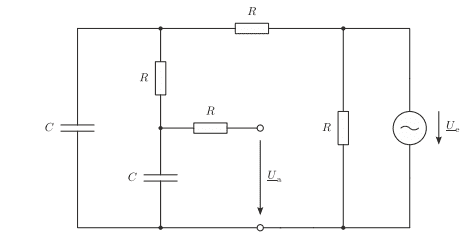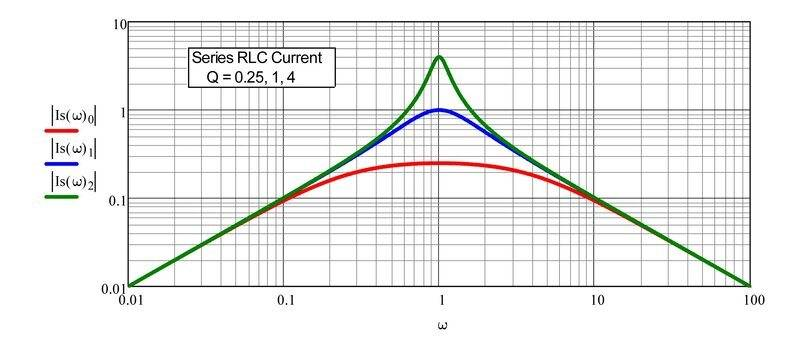# How do I draw the Bode diagram of this transfer function?

• Engineering
• arhzz
This would allow another engineer, who does know the value of his particular RC, to easily relabel the graph in Hertz for his case. In summary, the conversation discusses the calculation and analysis of a transfer function for a circuit, including the absolute value and phase of the transfer function, the type and order of the filter represented by the circuit, and the 3 dB limit frequency. The conversation also mentions the difficulty of drawing a bode diagram without knowing the RC value and suggests using normalization and interpolation to estimate intermediate values.f

#### arhzz

Homework Statement
Draw the bodediagram
Relevant Equations
-
Hello! I have this filter herea)
Calculate the transfer function T(Ω) = Ua/Ue using voltage dividers.For this, use the normalized angular frequency Ω = ωRC and bring the result into the form ##T(Ω) = \frac{A+jB}{C+jD} ## . The result must not contain any double fractions.

I was able to that I have redrawn the circuit and used a double voltage divider to get this result
$$\frac {1}{ 1 - Ω^2 + j3Ω}$$ and I think it should be correct

b)In general, calculate the absolute value |T(Ω)| and the phase arg(T(Ω)) of the transfer function. It must not be conjugated complex.

So for the absolute value I did this $$sqrt{\frac{1}{(1-Ω^2)^2+(3Ω)^2}}$$ since its only general. And for the phase I think it should be
##arg(T(Ω)) = arctan(\frac{Im}{Re}) - arctan(\frac{Im}{Re})## where the first arctan is the one from the nummerator and the second one from the denominator. Since the first one is 0 what remains is ; ## - arctan(\frac{3Ω}{1-Ω^2}) ##

c) What kind and order of filter does the above circuit represent?

It is a low pass , 2nd order

d) Calculate the 3 dB limit frequency Ωg with |T max| = |T(Ω → 0)|.

I was also able to this since $$|T(Ω)| = \frac{Tmax){\sqrt{2}}$$ and after solving it I get that Ωg = 0,37

Now up to this point I had no real issues and all of my solutions match the one from the book. But here is where I am kind of stumped

e) sketch the Bode diagram (magnitude and phase) as a function of the normalized angular frequencyΩ. Calculate the magnitude and phase of the transfer function for ̈Ω=[0.1, 0.4, 0.6, 0.8, 1, 2, 4,90]and interpolate the values in between.Continue to draw the asymptote in the diagramm.

Now I am not really sure how to do this. I mean I could just plug in the values for omega into the equations but I have no cutoff frequency. I know what a bode diagramm of a low pass filter looks like and how to calculate the cutoff frequency of one but I have no values for C and R. Can I draw it without the cutoff frequency? Also I am not sure how to interpolate the values in between (we have not done it before in class). For the asymptote I know what it is (at least what I've learned in mathematics) it is susposed to be the line that the graph approaches but never touches. I am guessing this will be apperent when I draw the graph.

So how should I approach this? Should I just plug in the values draw and see what kind of graph comes out? Disregarding the cutoff frequency?

First, sorry, I didn't check your work for the first parts; too busy. But it looks reasonable.

Homework Statement:: Draw the bodediagram
Relevant Equations:: -

Now I am not really sure how to do this. I mean I could just plug in the values for omega into the equations but I have no cutoff frequency. I know what a bode diagramm of a low pass filter looks like and how to calculate the cutoff frequency of one but I have no values for C and R. Can I draw it without the cutoff frequency?

As you said, you can't plot the response versus frequency without knowing the RC value. That's OK, it's built into the problem. You can just make a bode plot that has log(Ω) on the x-axis instead of log(f) [frequency]. We call this normalization, which can be useful to describe a whole class of problems. They've already defined a value that every frequency is referenced to, Ω=ωRC, so your frequency is f= Ω/(2πRC). Then put a label on your graph that states that definition. This would allow another engineer, who does know the value of his particular RC, to easily relabel the graph in Hertz for his case.

Here is an example for a different circuit (your Ω is the same as my ω).•arhzz
Interpolation is a straightforward method of estimating values between your data points. Unless someone says otherwise you can assume they mean matching a linear function to two data points and using that to find intermediate values. This should be easy to learn about with a web search. Research it and ask if there's something you find confusing in the explanation.

•arhzz
First, sorry, I didn't check your work for the first parts; too busy. But it looks reasonable.

As you said, you can't plot the response versus frequency without knowing the RC value. That's OK, it's built into the problem. You can just make a bode plot that has log(Ω) on the x-axis instead of log(f) [frequency]. We call this normalization, which can be useful to describe a whole class of problems. They've already defined a value that every frequency is referenced to, Ω=ωRC, so your frequency is f= Ω/(2πRC). Then put a label on your graph that states that definition. This would allow another engineer, who does know the value of his particular RC, to easily relabel the graph in Hertz for his case.

Here is an example for a different circuit (your Ω is the same as my ω).

View attachment 302584
Great answer! So now if I want the values of the diagramm (magnitude and phase) i can just plug in the given values in the formulas and when drawing the diagramm add the label that its normalized on the x axis?

•DaveE